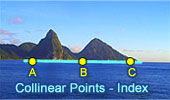# Collinear Points, Theorems and Problems - Table of Content 6Geometry Problem 631.
Triangle, Exterior Angle Bisectors, Collinear Points.

Geometry Problem 613
Altitude of a Triangle, Perpendicular, Collinear Points.

Geometry Problem 612
Altitude of a Triangle, Perpendicular, Congruence.

Geometry Problem 611
Altitude of a Triangle, Perpendicular, Collinear Points, Parallel.

Geometry Problem 609
Triangle, Perpendiculars, 90 Degrees, Collinearity.

Go to page:  Previous | 1 | 2 | 3 | 4 | 5 | 6 | 7 | 8 | 9 | 10 | Next